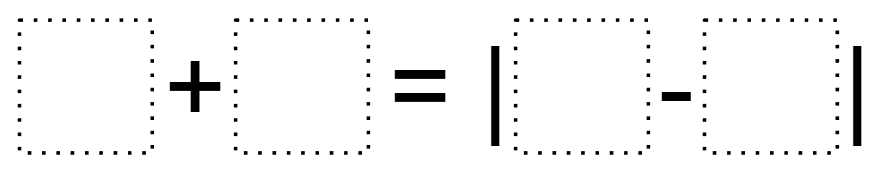Home > Grade 7 > Absolute Value 2

# Absolute Value 2

Directions: Using the digits 1 to 9 at most one time each, fill in the boxes to make the equality true:### Hint

What does absolute value mean? How does that relate to two numbers? How would that relate to equality?

There are many possible solutions. One example would be 3 + 4 = | 2 – 9 |

Source: Bryan Anderson

## Converting a Fraction to a Decimal

Directions: Using the digits 1 to 9, at most one time each, place a digit …

### One comment

1.I don’t find this problem SO worth solving, as the absolute value is not needed (the digits of the subtraction can be arranged in any order to create a positive value).

It would be much more interesting if the 7 selected digits were asked to be arranged from least to greatest in a sentence like this: ____ + ____ ____ = | ____ ____ – ____ ____ |